# ΔAmt∼δAhe, Construct δ Amt Such that Ma = 6.3 Cm, ∠Mat=120°, at = 4.9 Cm and "Ma"/"Ha"=7/5, Then Construct δAhe. - Geometry

Sum

ΔAMT∼ΔAHE, construct Δ AMT such that MA = 6.3 cm, ∠MAT=120°, AT = 4.9 cm and "MA"/"HA"=7/5, then construct ΔAHE.

#### Solution

Δ AMT and Δ AHE are give

⇒ ("MA")/("HA")= ("AT")/("AE") =("TM")/("EH")=7/5

⇒ ("MA")/("HA")=7/5 ⇒ 6.3/"HA" =7/5

⇒ "HA" =(6.3xx5)/7

HA = 4.5cm

Similarly, "AT"/"AE" =7/5

⇒ 4.9/"AE" =7/5 ⇒"AE" =(4.9xx5)/7⇒"AE"=3.5cm

Given triangle Required triangle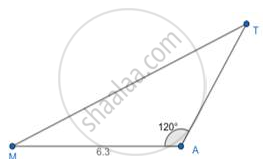Steps of Constructing the required triangle:

1. Draw a line segment of some length HP and mark an arc of 4.5cm(as calculated above) and name it as A.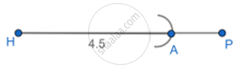2. At vertex A, make an angle of 120°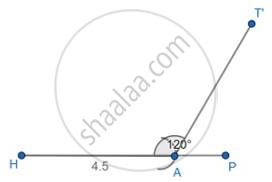3. Mark an arc of 3.5cm (as calculated above) on AT’ and name it E.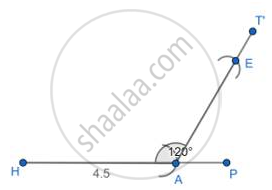4. Join HE.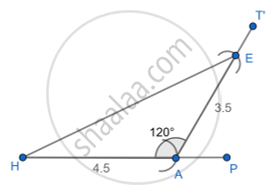5. Δ AHE is the required triangle.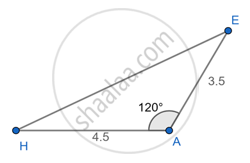Concept: Application of Trigonometry
Is there an error in this question or solution?